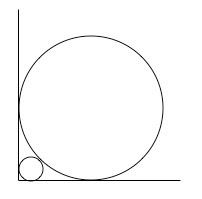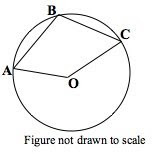## Monday, July 28, 2008

### Taking Two or More At a Time- What is the Probability You Don't Like These!

Only a few days left for your July MathAnagram. Either it's harder than I thought or no one is paying attention!

SIR "OY", E. NOET!
Look here for details.

Probability questions will forever addle the minds of students and adults alike. If all problems could involve selecting one object randomly, life would be good. Unfortunately, selecting 'more than one' is becoming common on standardized tests these days. Taking two or more objects immediately ratchets up the difficulty:

Does order count?
Multiple solution paths
Making a list
Combinations? Permutations? Multiplication Principle? Using "rules" of probability?

In what course do students receive sufficient instruction in this important area? Algebra 1? Algebra 2? Precalculus? A probability/statistics/discrete math class? AP Stat? IMO, the lack of standardization in secondary curriculum can lead to some topics getting short shrift.

I've come to the conclusion that middle schoolers should devote more time to some of this, since 4th graders are generally expected (in most states' standards) to solve the "select one object" type. What do you think? Since most readers enjoy the math challenges and not this kind of curriculum discussion, here are our offerings for today...

A bag contains eight coins: two each of pennies, nickels, dimes and quarters.

Question 1: If two coins are randomly selected, show that the probability that the two coins will total at least 20 cents in value is 1/2.

Question 2: If 4 coins are randomly selected, show that the probability of getting exactly one of each kind of coin is 8/35. (At least two methods please!)
Note: This result implies that the chances of getting at least one matching pair of coins among the 4 coins is greater than 75%!!

There are many problems of this type on this blog (see probability, combinatorial math in the index). Further, there are many other excellent blogs and web sites that address these topics and provide wonderful challenges and explanations. Two of the best are Jonathan's over at jd2718 and Isabel over at God Plays Dice.

## Wednesday, July 23, 2008

### Everything's a Square! Motivating Students to Use Deductive Reasoning

Hi y'all! Enjoying a restful peaceful summer? Just a few thoughts...

1. Only one correct submission to this month's math anagram"

SIR "OY", E. NOET!

Please follow the rules here and email me by the end of the month.

2. Ok, so what's going on with the 3 "squares" in the above diagram? In a recent post, we looked at using "Figure Not Drawn To Scale" as an effective way to encourage student reasoning and to become more cautious about making assumptions. Making a variety of quadrilaterals all "appear" to be squares as in the above diagrams is consistent with this approach.

Even before teaching the formal theorems and definitions regarding quadrilaterals in "Chapter 5" of the text, why not begin with a preview activity? If you prefer to wait until students have the necessary definitions, theorems and postulates, then one can use this as an application. Your choice...

Suggested Questions:
Does the given information in each diagram guarantee that each is a square?
If you don't think so, your mission is to draw a quadrilateral with the given information but clearly does NOT look like a square.
Alright, think about the first one. After a minute share your thoughts, diagrams with your partner. Go!
Etc...

If using this to review the standard definitions and theorems on quadrilaterals, I would still encourage the drawing of diagrams to illustrate that the first two figures do not have to be squares.

## Monday, July 14, 2008

### Squeezing Circles Into the Corner: An Infinite Sequence Investigation in GeometryAnother summer diversion from geometry...

The number of variations for tangent circles is endless and this is one of my all-time favorites. Math contests and SATs seem to have a preference for circles inscribed in squares or tangent circle problems and this one is along those lines. However, the real payoff comes from developing recursive thinking leading to an infinite geometric sequence and its sum! Students will be asked to intuitively "guess" the value of this infinite sum and to then verify their conjecture. Proving it requires nothing more than the classic formula for the sum of an infinite geometric series but, at the outset, this problem is eminently suitable for your geometry classes. Don't hesitate to use it in your "regular" classes. Questions that are deemed appropriate only for honors classes are often suitable for most students if the groundwork is laid (background, examples, etc.) and hints are given strategically.

PART I In the diagram above the larger circle has radius 1, the two circles are tangent to each other and to the two perpendicular segments (you can think of the larger circle being inscribed in a square if you wish).

(a) Make a conjecture from the diagram without computing: The ratio of the radius of the smaller circle to the larger is approximately

(A) 0.05 (B) 0.15 (C) 0.25 (D) 0.35 (E) 0.5
Note: This part may be omitted.

(b) Show that the radius of the smaller circle is exactly (√2 - 1)2 = 3 - 2√2
Note: Your decision about giving them the result like this. Obviously if they see part (b) on a worksheet, their estimate in part (a) will be pretty good! My intent was to focus on the method. Of course, feel free to rephrase this.

PART II
Of course we will not stop at 2 circles! Squeeze a third circle into the corner between the 2nd circle and the right angle. Determine its radius by using the result from part (a). [The key here is to think ratios!]

PART III
If we label the radius of the largest circle R1, the radius of the 2nd circle R2, the radius of the 3rd circle R3, etc., we can now define an infinite sequence of these radii.
(a) Find a formula for the nth term of this sequence, n = 1,2,3,..

(b) What is the mathematical terminology for this type of sequence?

(c) Think intuitively here: From the diagram, what should be the "sum" of the original radius R1 = 1 and the diameters of the remaining infinite collection of circles. [Another formulation: As n-->∞, this sum approaches what number?]

(d) Using the formula for the sum of an infinite geometric series, verify your conjecture in (c).

• As always, feel free to use this with your students and revise as you see fit. However, pls use the attribution in the Creative Commons License as indicated in the sidebar.
• Finding the radius of the 2nd circle is a challenge by itself and the problem could stop there. The extensions can be assigned as a long-term project or for those wishing to do extra credit. I always liked having additional challenges for the students who were capable of going further, although relating this problem to geometric sequences or series is of importance. Of course, I am well aware of time constraints faced by the instructor.

## Wednesday, July 9, 2008

### "Any Way You Slice It" - A Classic Cube Dissection Problem to the Nth!

The following series of questions was inspired by a recently released SAT question. The first two levels are appropriate for middle or secondary students. Level III requires more algebraic background or strong visualization skills. I could have attempted to include a graphic for some of this but I'll leave that to the experts out there!

LEVEL I
A cube is cut into 8 equal cubes by dividing each edge in half with three planes which are parallel to the faces of the original cube. Show that the total surface area of the 8 smaller cubes (when separated) is TWICE the surface area of the original cube.

Note: Most secondary students would attempt this algebraically or substitute particular values. To develop spatial sense, encourage them to find another solution, which is purely visual and elegant! Middle school students (or younger children) would greatly benefit from constructing a physical model of the cube from modeling clay (or something equivalent) and slicing it with appropriate tools. Better yet, one can avoid slicing by constructing the bigger cube from 8 smaller cubical blocks (There are many sets of plastic or wooden blocks available from catalogs).

LEVEL II
A cube is cut into 27 equal cubes by dividing each edge into 3 equal parts with planes parallel to the faces of the original cube. Show that the total surface area of the 27 cubes is THREE times the surface area of the original cube.

LEVEL III
Generalize the above relationships by dividing each edge of a cube into N equal parts with planes parallel to the faces of the original cube (N is an integer greater than 1). State a conclusion and explain! Again, try to find both an algebraic and a visual explanation.

## Monday, July 7, 2008

### Figure Not Drawn To Scale! An SAT-Type Geometry/Summer DiversionIn the circle at the left, O is the center, A, B and C are on the circle and OABC is a parallelogram. If AB = 6, what is the length of segment AC (not drawn)?

(A) 3√2 (B) 3√3 (C) 6 (D) 6√2 (E) 6√3

POINTS TO PONDER

Is this an appropriate standardized test question?

Are you an opponent of multiple choice (aka, "multiple guess") questions. Why?

We can also say much about the issue of drawing figures that do not appear to be what they are? Is it just the testmaker's way of misleading or trapping students or is there a valid purpose to this?